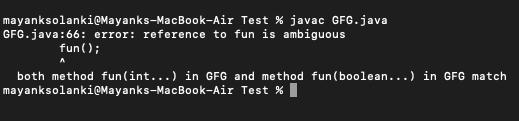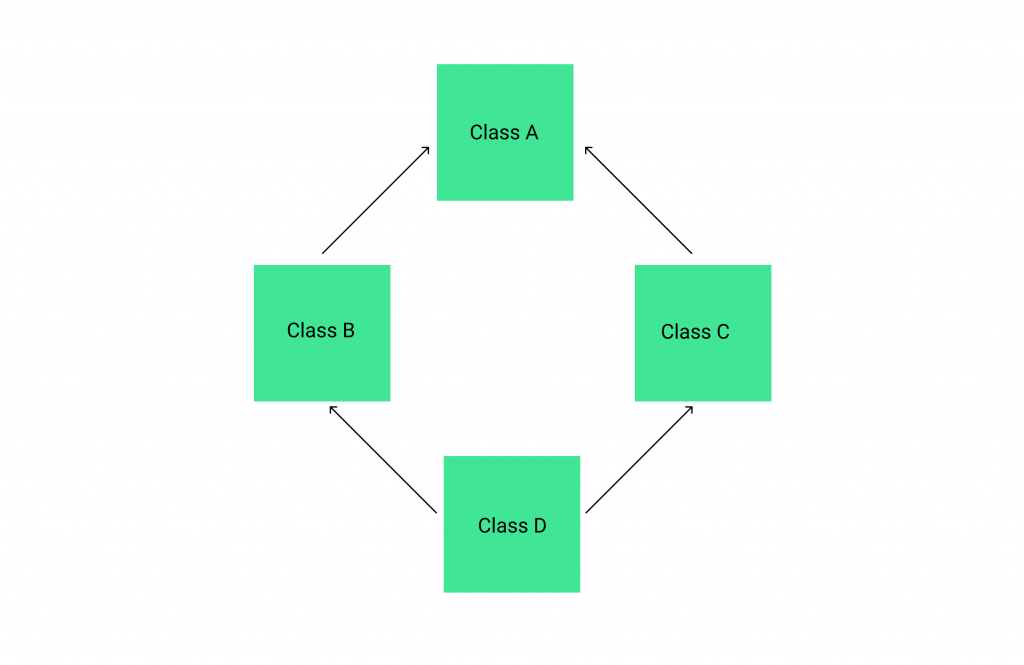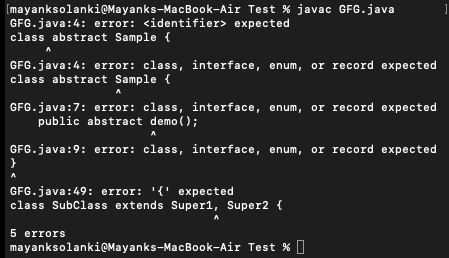Related Articles

# Ambiguities in Java

• Difficulty Level : Medium
• Last Updated : 10 Sep, 2021

The ambiguities are those issues that are not defined clearly in the Java language specification. The different results produced by different compilers on several example programs support our observations. Here we will be discussing in the following order.

• Methods with only Varargs parameters
• Methods with Varargs along with other parameters
• Ambiguity in multiple inheritances
• Diamond Problem

When you overload methods, you risk creating an ambiguous situation of which one is in which the compiler cannot determine which method to use. For example, consider the following overloaded computeBalance() method declarations:

`public static void computeBalance(double deposit)`
`public static void computeBalance(double withdrawal)`

If you declare a double variable named myDeposit and make a method call such as computeBalance(myDeposit);, you will have created an ambiguous situation. Both methods are exact matches for your call. You might argue that a call using a variable named myDeposit “seems” like it should go to the version of the method with the parameter named deposit, but Java makes no assumptions based on variable names. Each version of computeBalance() could accept a double, and Java does not presume which one you intend to use.

Ambiguity Errors

The inclusion of generics gives rise to a new type of error that you must guard against ambiguity. Ambiguity errors occur when erasure causes two seemingly distinct generic declarations to resolve to the same erased type, causing a conflict. Here is an example that involves method overloading.

Now we are good to go with type 1 as shown above to describe that is. method overloading in varargs

Overloading allows different methods to have the same name, but different signatures where the signature can differ by the number of input parameters or type of input parameters, or both. We can overload a method that takes a variable-length argument by following ways:

Case 1: Methods with only Varargs parameters

In this case, Java uses the type difference to determine which overloaded method to call. If one method signature is strictly more specific than the other, then Java chooses it without an error.

Example

## Java

 `// Java program to illustrate method overloading in varargs` `// Main class demonstrating varargsDemo``public` `class` `GFG {` `    ``// Method 1``    ``// varargs method with float datatype``    ``static` `void` `fun(``float``... x)``    ``{` `        ``// Print statement``        ``// whenever this method is called``        ``System.out.println(``"float varargs"``);``    ``}` `    ``// Method 2``    ``// varargs method with int datatype``    ``static` `void` `fun(``int``... x)``    ``{` `        ``// Print statement``        ``// whenever this method is called``        ``System.out.println(``"int varargs"``);``    ``}` `    ``// Method 3``    ``// varargs method with double datatype``    ``static` `void` `fun(``double``... x)``    ``{` `        ``// Print statement``        ``// whenever this method is called``        ``System.out.println(``"double varargs"``);``    ``}` `    ``// Method 4``    ``// Main driver method``    ``public` `static` `void` `main(String[] args)``    ``{` `        ``// Calling the above methods``        ``fun();``    ``}``}`
Output
`int varargs`

Output Explanation:

This output is due to the fact that int is more specific than double. As specified in the JLS section 15.12.2.5, If more than one member method is both accessible and applicable to a method invocation, it is necessary to choose one to provide the descriptor for the run-time method dispatch. The Java programming language uses the rule that the most specific method is chosen according to type promotion. The following rules define the direct supertype relation among the primitive types in this case:

```double > float
float  > long
long   > int
int    > char
int    > short
short  > byte```

Case 2: Methods with Varargs along with other parameters. In this case, Java uses both the number of arguments and the type of arguments to determine which method to call.

Example 1:

## Java

 `// Java program to demonstrate Varargs and Overloading` `// Main class``class` `GFG {` `    ``// Method 1``    ``// It takes varargs(here integers).``    ``static` `void` `fun(``int``... a)``    ``{` `        ``// Print statement whenever this method is called``        ``System.out.print(``"fun(int ...): "``                         ``+ ``"Number of args: "` `+ a.length``                         ``+ ``" Contents: "``);` `        ``// For each loop is used to``        ``// display contents``        ``for` `(``int` `x : a)` `            ``// Print statement``            ``System.out.print(x + ``" "``);` `        ``// New line``        ``System.out.println();``    ``}` `    ``// Method 2``    ``// It takes varargs(here booleans).``    ``static` `void` `fun(``boolean``... a)``    ``{` `        ``// Print statement to display the content``        ``// whenever this method is called``        ``System.out.print(``"fun(boolean ...) "``                         ``+ ``"Number of args: "` `+ a.length``                         ``+ ``" Contents: "``);` `        ``// Iterating using for-each loop to``        ``// display contents``        ``for` `(``boolean` `x : a)` `            ``// Print statement to display the content``            ``// whenever this method is called``            ``System.out.print(x + ``" "``);` `        ``// New line for better readability``        ``System.out.println();``    ``}` `    ``// Method 3``    ``// It takes string as a argument``    ``// followed by varargs(here integers).``    ``static` `void` `fun(String msg, ``int``... a)``    ``{` `        ``// Print statement to display the content``        ``// whenever this method is called``        ``System.out.print(``"fun(String, int ...): "` `+ msg``                         ``+ a.length + ``" Contents: "``);` `        ``// Iterating using for-each loop to``        ``// display contents``        ``for` `(``int` `x : a)` `            ``System.out.print(x + ``" "``);` `        ``// New line for better readability``        ``System.out.println();``    ``}` `    ``// Method 4``    ``// Main driver method``    ``public` `static` `void` `main(String args[])``    ``{` `        ``// Calling the above methods to``        ``// check for overloaded fun()``        ``// with different  parameter` `        ``// Custom inputs as parameters` `        ``fun(``1``, ``2``, ``3``);``        ``fun(``"Testing: "``, ``10``, ``20``);``        ``fun(``true``, ``false``, ``false``);``    ``}``}`
Output
```fun(int ...): Number of args: 3 Contents: 1 2 3
fun(String, int ...): Testing: 2 Contents: 10 20
fun(boolean ...) Number of args: 3 Contents: true false false ```

Here the fun() method is overloaded three times.

Note: Sometimes unexpected errors can result when overloading a method that takes a variable-length argument. These errors involve ambiguity because both the methods are valid candidates for invocation. The compiler cannot decide on which method to bind the method call.

Example 2:

## Java

 `// Java program to illustrate Varargs and Ambiguity` `// Main class``class` `GFG {` `    ``// Method 1``    ``// It takes varargs(here integers).``    ``static` `void` `fun(``int``... a)``    ``{` `        ``// Print and display contents``        ``// whenever this method is called``        ``System.out.print(``"fun(int ...): "``                         ``+ ``"Number of args: "` `+ a.length``                         ``+ ``" Contents: "``);` `        ``// Iterating using for-each loop to``        ``// display contents``        ``for` `(``int` `x : a)` `            ``// Print statement``            ``System.out.print(x + ``" "``);` `        ``// New line for better readability of output``        ``System.out.println();``    ``}` `    ``// Method 2``    ``// It takes varargs(here booleans).``    ``static` `void` `fun(``boolean``... a)``    ``{` `        ``// Print and display contents``        ``// whenever this method is called``        ``System.out.print(``"fun(boolean ...) "``                         ``+ ``"Number of args: "` `+ a.length``                         ``+ ``" Contents: "``);` `        ``// Iterating using for-each loop to``        ``// display contents``        ``for` `(``boolean` `x : a)` `            ``System.out.print(x + ``" "``);` `        ``// New line is needed for``        ``// better readability in output``        ``System.out.println();``    ``}` `    ``// Method 3``    ``// Main driver method``    ``public` `static` `void` `main(String args[])``    ``{` `        ``// Calling overloaded fun() above created``        ``// with different  parameter` `        ``// Custom inputs are passed as parameters` `        ``// Case1: ok``        ``fun(``1``, ``2``, ``3``);` `        ``// Case 2: ok``        ``fun(``true``, ``false``, ``false``);` `        ``// Case 3: Error: Ambiguous!``        ``fun();``    ``}``}`

Output:Output explanation: The overloading of the desired method here named ‘fun()‘ is perfectly correct. However, this program will not compile because of the last call made to ‘fun()’ which can also be interpreted in the code.

Type 2: Ambiguity in multiple inheritances. Inheritance is a relation between two classes where one class inherits the properties of the other class. This relation can be defined using the extends keyword as follows:

`public class A extends B {}`

The class which inherits the properties is known as a subclass or, child class and the class whose properties are inherited is a superclass or, parent class. In inheritance, a copy of superclass members is created in the sub-class object. Therefore, using the sub-class object you can access the members of both classes.

There are various types of inheritance available namely single, multilevel, hierarchical, multiple and, hybrid. In multiple inheritances, one class inherits the properties of multiple classes. In other words, in multiple inheritances, we can have one child class and n number of parent classes. Java does not support multiple inheritances (with classes).

Implementation:

Diamond problem is one of the major ambiguities that arise here in the case of multiple inheritances. For instance, let us assume that Java does support multiple inheritances. Consider the example below with the following assumptions. Here we have an abstract class named ‘Sample‘ with an abstract method asExample

## Java

 `// Java Program to illustrate Diamond Problem Ambiquity` `// Class 1``// Abstract class``// Parent class``public` `class` `abstract` `Sample {` `    ``// Abstract method``    ``public` `abstract` `demo();``}` `// Then in the same package/folder,``// we have two classes extending this class and``// trying to implement its abstract method, demo().` `// Class 2``// helper class extending Class 1``public` `class` `Super1 ``extends` `Sample {` `    ``// Method of this base class``    ``public` `void` `demo()``    ``{` `        ``// Print statement whenever this method is called``        ``System.out.println(``"demo method of super1"``);``    ``}``}` `// Class 3``// Helper class extending Class 1``public` `class` `Super2 ``extends` `Sample {` `    ``// Method of this base class``    ``public` `void` `demo()``    ``{` `        ``// Print statement whenever this method is called``        ``System.out.println(``"demo method of super2"``);``    ``}``}` `// According to our assumption of Java supports multiple``// inheritance we are trying to inherit both classes Super1``// and Super2.` `// Class 4``// Helper class``// Deriving above two classes: Class2 and Class3``public` `class` `SubClass ``extends` `Super1, Super2 {` `    ``// Method of this class``    ``// Also, it is main driver method``    ``public` `static` `void` `main(String args[])``    ``{` `        ``// Creating object``        ``SubClass obj = ``new` `SubClass();` `        ``// Trying to access the demo() method``        ``// with the help of this class object``        ``obj.demo();``    ``}``}`

Output:Output Explanation: Then, as per the basic rule of inheritance, a copy of both demo() methods should be created in the subclass object which leaves the subclass with two methods with the same prototype (name and arguments). Then, if you call the demo() method using the object of the subclass compiler faces an ambiguous situation not knowing which method to call. This issue is known as the diamond problem in Java.

Attention reader! Don’t stop learning now. Get hold of all the important Java Foundation and Collections concepts with the Fundamentals of Java and Java Collections Course at a student-friendly price and become industry ready. To complete your preparation from learning a language to DS Algo and many more,  please refer Complete Interview Preparation Course.

My Personal Notes arrow_drop_up